# Understanding Statistical Arbitrage: A Path to Profitable Trading Wednesday June 21st, 2023 – Posted in: Arbitrage Software, cryptoarbitrage software – Tags: arbitrage software, forex arbitrage, statistical arbitrage, statistical arbitrage bot, statistical arbitrage software

Statistical arbitrage, often called “stat arb,” is a popular quantitative trading strategy widely employed by hedge funds and proprietary trading firms. The fundamental concept involves exploiting pricing inefficiencies between related financial instruments. Traders leveraging stat arb rely on complex mathematical models to identify trading opportunities, making this strategy an aspect of algorithmic trading.

### Origins of Statistical Arbitrage

Statistical arbitrage had its roots in the 1980s when it was pioneered by Wall Street’s quantitative analysts, colloquially known as ‘quants.’ The strategy was initially applied within equities markets, where pairs of stocks were selected based on their co-integration – a statistical property indicating that the price gap between the two stocks is mean-reverting over time. Since then, stat arb has evolved and been adapted to various other markets, including forex and cryptocurrencies.

### The Core Principle

Statistical arbitrage relies on mean reversion principles and the law of large numbers. The underlying belief is that the relative prices of financial instruments that are historically correlated will revert to their mean over time. This is where statistical arbitrage occurs – it capitalizes on price discrepancies between these correlated instruments when they deviate from their historical norm.

For instance, consider two stocks that have moved together historically. If their prices diverge – one increases in price, and the other decreases – a statistical arbitrageur would sell short the outperforming stock and buy the underperforming one, betting that the “spread” between the two would eventually converge.

### Spread – to the price difference or discrepancy between two related financial instruments.

In statistical arbitrage, the term ‘spread’ typically refers to the price difference or discrepancy between two related financial instruments. These could be two different stocks, futures contracts, forex pairs, or even cryptocurrency tokens.

For example, one might track the spread between two historically co-integrated stocks in a pair trading strategy (a common form of statistical arbitrage). When the spread, or the difference in their prices, deviates significantly from its historical mean (average), it signals an opportunity to trade.

Suppose the spread widens too much, indicating one stock is overpriced and the other is underpriced relative to their historical relationship. In that case, a trader may sell short the overpriced stock and buy the underpriced one. Conversely, if the spread narrows excessively, they would do the opposite.

The spread is expected to be mean-reverting in statistical arbitrage, meaning it fluctuates around a long-term average value. Traders expect that when the spread deviates significantly from this mean, it will eventually return to it, allowing them to profit from this reversion.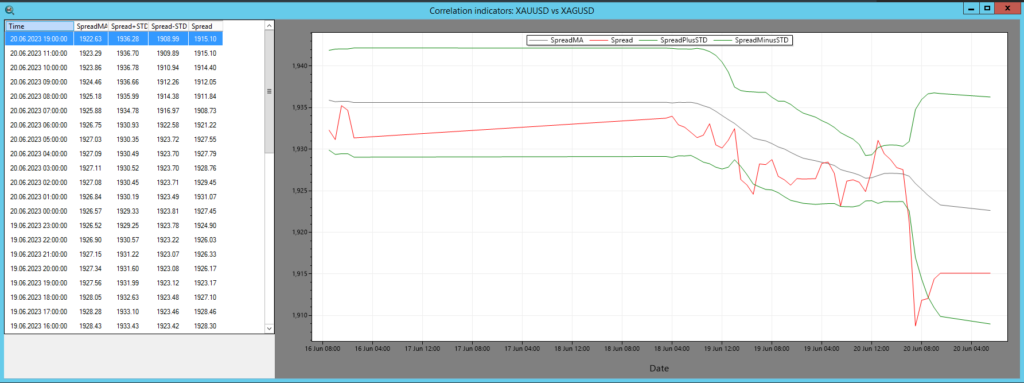In the SharpTrader™ Arbitrage software, the Spread Indicator is utilized to visualize the correlation between two assets. The calculation of the correlation involves the following components:

1. Spread: This refers to the numerical difference or distance between the values of two assets.
2. SpreadMA: It represents the moving average of the spread over a specific period, determined by pi_SpreadMA_Period.
3. STD (Standard Deviation): It calculates the classic standard deviation of the spread relative to SpreadMA. The number of observations used is equal to pi_SpreadMA_Period.

To trigger the opening of trades, the software follows the principles of statistical arbitrage theory.

### A little bit of theory about correlation calculation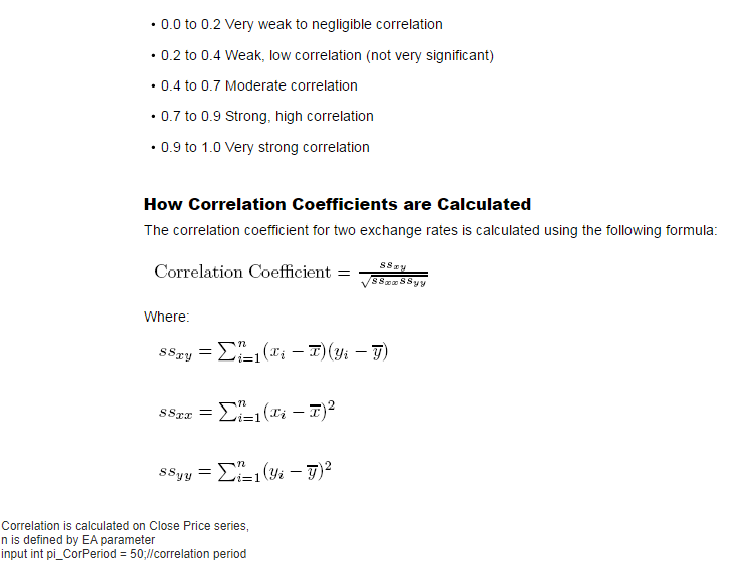### Statistical arbitrage – correlation matrix

A correlation table, also known as a correlation matrix, is a table that shows the correlation coefficients between many variables. Each cell in the table shows the correlation between two variables. The value is in the range of -1 to 1.

If two variables have a correlation of 1, they move in the same direction, i.e., when one increases, the other increases, and vice versa. This is known as a perfect positive correlation.

If two variables correlate -1, they move in opposite directions, i.e., when one variable increases, the other decreases, and vice versa. This is known as a perfect negative correlation. A correlation of 0 means that no relationship exists between the variables.

Here’s a simple example of a correlation table for three variables: A, B, and C: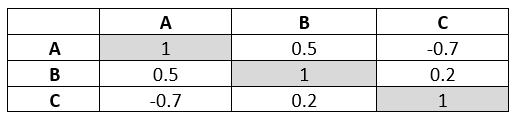Pic. 2 – Example of correlation table

In this table, the correlation between variables A and B is 0.5 (a moderate positive correlation), while the correlation between A and C is -0.7 (a strong negative correlation). The diagonal of the matrix from the top left to the bottom right is always 1 because a variable is perfectly correlated with itself.

These tables are widely used in various fields, including finance, where they help in determining the relationship between different financial variables or the returns of different assets, useful for portfolio diversification and risk management.

You can generally create this correlation matrix using statistical software or programming languages like Python or R. Here’s a simple way to do it with Python using the pandas and numpy libraries:

import pandas as pd

import numpy as np

# Assuming you have historical data in two lists

crude_oil_prices = […]

texas_oil_prices = […]

# Create a DataFrame

df = pd.DataFrame({‘Crude Oil’: crude_oil_prices, ‘Texas Oil’: texas_oil_prices})

# Calculate the correlation matrix

correlation_matrix = df.corr()

print(correlation_matrix)

This script will output a 2×2 correlation matrix with the correlation coefficients between crude oil and Texas oil prices.

## Implementing the Statistical Arbitrage Strategy

The implementation of statistical arbitrage is a complex task. It requires sophisticated statistical tools and models, high-speed computational systems, and advanced algorithms. The approach involves:

• Pair Selection: The first step is identifying pairs of assets that are historically correlated. This is typically done using statistical methods like co-integration tests.
• Threshold Determination: Next, traders establish upper and lower thresholds for divergence. Asset prices deviate beyond these thresholds, triggering a trading signal.
• Trade Exit: When the prices of the two assets converge (i.e., the spread reverts to the mean), the positions are closed.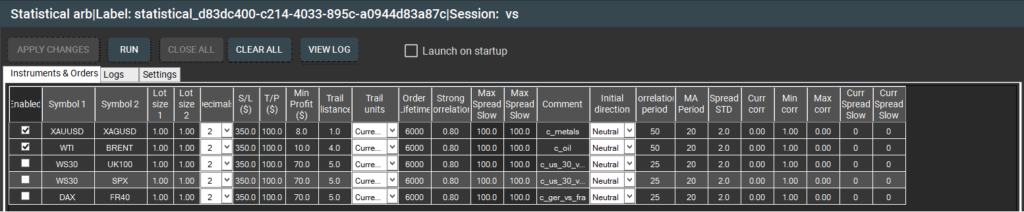Pic. 3 – SharpTrader™ Statistical arbitrage built-in strategy “Instruments & Orders” window.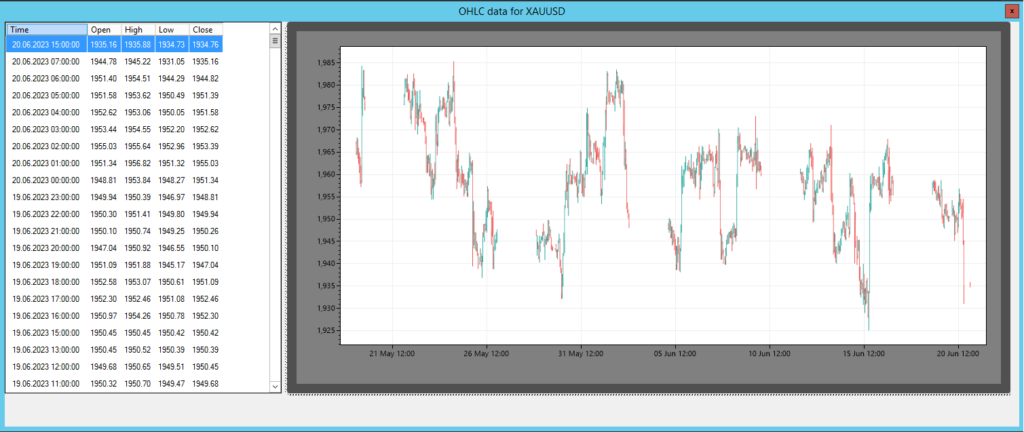Pic. 4 – SharpTrader™ Statistical Arbitrage quotes and graphs

#### Risks and Limitations

Like any trading strategy, statistical arbitrage is not without risk. One of the most significant risks is “model risk” – the potential for the mathematical models to be based on invalid assumptions.

Moreover, the strategy’s success also heavily relies on the speed of execution, as price inefficiencies can disappear in milliseconds. This reliance on speed approaches is more suited to algorithmic trading systems than individual traders.

### The Future of Statistical Arbitrage

With financial markets becoming more efficient and automated, the future of statistical arbitrage lies in developing more sophisticated algorithms and models, machine learning, and artificial intelligence. As the pace of technological innovation continues, so will the evolution of statistical arbitrage.

In conclusion, statistical arbitrage, with its complex mathematical models and reliance on high-speed execution, offers a technologically advanced approach to trading. Despite the challenges and risks, it remains an attractive strategy due to its potential for consistent, low-risk profits.

The application of Artificial Intelligence (AI) in statistical arbitrage has opened up new avenues to enhance trading strategies and improve their predictive accuracy. Machine learning, a subset of AI, is especially useful in identifying complex patterns and making forecasts based on large datasets. Here’s how you can use AI for statistical arbitrage:

Data Collection: Gather historical price data of the financial instruments you’re interested in. This data might include open, high, low, and close prices and trading volumes. It could also incorporate relevant macroeconomic data, such as interest rates or GDP figures if these are considered significant for the traded assets.

#### Feature Engineering

Use this data to create a set of features (input variables) for your AI model. This could involve calculating technical indicators, such as moving averages or RSI, or creating variables representing the price difference or ratio between pairs of assets.

#### Model Training

Feed these features into a machine learning model, such as a neural network or support vector machine, to predict future price movements or spread between your assets. The model will learn from the patterns in your historical data.

#### Model Validation

Validate your model’s performance using out-of-sample data. This data not used during the training process will give you a better understanding of how your model will perform in live trading.

Trade Signals: Use the model to generate trade signals. For instance, if the model predicts the narrow spread between two assets, you might buy the underperforming asset and sell the outperforming one short.

#### Risk Management

Always keep a risk management strategy in place. AI models, like all trading strategies, could be better and can make incorrect predictions. Limiting your risk on each trade and setting up stop losses is crucial.

Model Updating

Continuously monitor your model’s performance and update it with fresh data. The financial markets are dynamic, and a model that performed well in the past may only sometimes do well in the future. Regular updates and adjustments are crucial to maintaining the model’s performance. Remember, while AI can enhance your trading strategy, it’s not a silver bullet and doesn’t guarantee success.